# VBA ConstArticle byExcelMojo Team## What is VBA Const (Constants)

Const stands for Constants and is a storage box available in VBA. It is used to define a variable with a constant method and assign it a value that will always be constant.

VBA Const helps us to write codes where someone cannot change the value assigned to a constant variable. Consider a situation where we must use a person’s name in a macro several times. With a Const, we do not have to write the person’s name each time in double quotes; instead, we assign the person’s name to a constant variable, and we can use the constant variable throughout the macro. So, if we must change the name, it can be changed at a single point, i.e., at the step of assigning a value to the constant variable.

For example, look at the following code.

In this code, the constant variable “MyName” is of data type “String,” and we have assigned it the value “Peter” and printed the result using a message box.

The assigned value cannot be changed during the execution of the macro.

###### Key Takeaways
• VBA Const defines a variable when we want to maintain the same variable value throughout the procedure.
• Here, Const stands for Constants.
• Once a value is set to the VBA Const variable, we cannot reassign it another value.
• We can make the VBA Const available across all modules by declaring them with the “Public” key word.

### Syntax of Const Statement in VBA

Following is the general syntax of VBA Const.

Const [Name of the variable] As [Data Type to be Assigned] = [Value to be assigned to the defined variable]

• Const: This is the keyword used to define a constant variable.
• Name of the variable: We enter the variable name after the VBA Const keyword. All the rules of naming a variable are applicable here as well.
• Data Type: We must assign the data type that can be assigned to the defined variable. We can assign VBA data types like Int, Boolean, Long, String, etc.
• Variable Value: Once the variable declaration is made via VBA Const, in the same line, we will assign a value to the defined variable by entering an equal sign.

#### Condition of Constants in VBA

There are a few essential things we must keep in mind while we define a constant variable, and those are.

1. The name given to the VBA Const can contain a maximum of 256 characters.
3. We cannot use any of the VBA keywords to name the VBA Const variable name.
4. The name should not contain space characters and special characters. However, we can use only underscore characters, for example, Total_Value.
5. We can declare multiple constants with a single VBA Const statement.

In VBA, we can declare two types of constants. They are:

• Intrinsic Constants: These are the constants built into the VBA language system. For instance, vbOk, vbOkCancel, vbOkOnly, etc., are some of the intrinsic constants.
• User-Defined Constants: These are the constants the user defines using the VBA Const.

### How to Use VBA Const?

Let us show you a step-by-step approach to defining a VBA Const variable.

1. Start the macro by naming the subroutine procedure.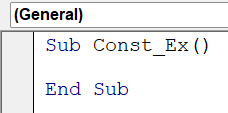2. To define a constant variable, use the Const keyword.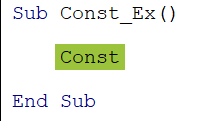3. Now, enter the variable name per the conditions above.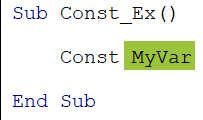4. Once the variable name is given, we must assign it a data type. We can assign any data type to the defined variable based on the requirement. For example, we assign the long data type to the variable.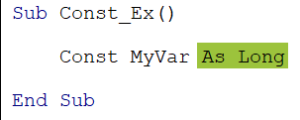5. Usually, the declaration of a variable ends here. However, when we declare a constant variable, we must go one step further and assign a value to the defined variable after the equal sign.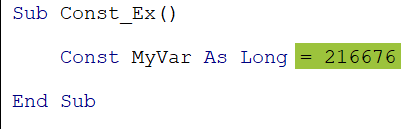Now we can use this constant variable “MyVar” within this sub-routine procedure, and the value of this variable (216676) remains the same throughout the sub-procedure; we cannot reassign any other value to the variable.

### Examples of Const Statement in VBA

Let us show you some examples of VBA Const variables.

#### Example #1 – Define a Constant and Assign a Value to It

Assume we are calculating the incentives for some salespeople in an Excel worksheet.

The incentive will be 5% of the sales value, where the 5% remains constant. Hence, we will assign this 5% value to a constant variable.

The following code is an example of the same.

Sub Const_Example1()
‘Define a variable and find the last used row
Dim LR As Long
LR = Cells(Rows.Count, 1).End(xlUp).Row
‘Define a variable and find the last used column
Dim LC As Long
LC = Cells(1, Columns.Count).End(xlToLeft).Column
‘Define a variable to loop thorugh all cells
Dim k As Long
‘Define constant variable and the incentive %
Const Sales_Incentive As Variant = 0.05
MsgBox Sales_Incentive
‘Loop through all the cells
For k = 2 To LR
Cells(k, 3).Value = Cells(k, 2).Value * Sales_Incentive
Next k
End Sub

• In the above code, we have defined a constant variable Sales_Incentive.
• To this variable, we have assigned an incentive percentage of 5%.
• This value is multiplied by the sales value by looping through all the cells and gives us the incentive value in each cell of looping cells.
• When we run this code, we get the following output in the worksheet.
• As we can see in column “C,” we have got the incentive amount based on the constant variable (Sales_Incentive) value 0.05 (5%).

#### Example #2 – We Cannot Change the Assigned Value of a Constant Variable

One of the key features of the Dim statement variable is that we can assign it a given data type value at any stage of the sub-procedure, and we can assign any value of the same data type multiple times in the same sub-procedure.

For example, look at the following code.

Sub Const_Example2()
‘First Set of value to the variable
Dim MyVar As Long
MyVar = 1000
MsgBox MyVar
‘Second set of value to the variable
MyVar = 5000
MsgBox MyVar
End Sub

Part #1

• In this part, we define the variable “MyVar” and assign the data type “Long” to it.
• We assign the value “1000” to it and show the assigned value in the message box.
• Once we run the code, the following message box shows the part #1 assigned value.

Part #2

• In this part, we assign a different value to the same variable but the same data type value.
• In this stage, we assign a value of “5000” and get the following value in the message box.
•   We have assigned two different values of the same data type for the same variable, and it still accepts two different values.
• However, this is not the case with the VBA Const variable. Once the value is assigned to the variable, we cannot reassign any value.

For example, look at the following code.

Sub Const_Example2()
‘First Set of value to the variable
Const MyVar As Long = 1000
MsgBox MyVar
‘Second set of value to the variable
MyVar = 5000
MsgBox MyVar
End Sub

In Part #1, we have defined a variable with the VBA Const keyword and assigned a value “1000” to it.

In Part #2, for the same variable, we are reassigning a value “5000.” Let’s try to execute the code and see what happens.

We get a compiler error, “Assignment to constant not permitted.” It means we cannot reassign the value to the Const Variable once it is assigned. Once the value is assigned to the VBA Const variable, we cannot reassign it.

### How to Make Constants Available Across Modules?

When we define a VBA Const variable, it is available within that sub-procedure. However, they can be made available across all the modules. For this, we follow the below steps.

#### Step #1 – Declare VBA Const Variable to Make Them Available to One Module

To make the VBA Const variables available within the module, we must declare them at the top. For example, look at the following code.

In “Module 2” in the coding window, before we start the sub-procedure, we have defined VBA Const variables and assigned values to them accordingly.

In the subsequent sub-procedures, we have used these VBA Const variables. As we can see in the image, we have the VBA Cons String variable “First_Name” in the first sub-procedure and the second sub-procedure.

#### Step #2 – Declare VBA Const Variable to Make Them Available to All Modules

One of the drawbacks of the previous step, VBA Const variable declaration, is that we cannot use the defined variables in another module. For example, look at the following code.

We are in Module 1, and from here, we are trying to access the VBA Const variables defined in Module 2. As observed, the IntelliSense list doesn’t show up in the VBA Constants List defined in Module 2.

To make them available across modules, we must use the “Public” keyword while defining them. We use VBA Const Public to define the variable. For example, look at the following code.

In Module 2, we used the “Public” keyword to define a VBA Const variable.

Now let’s access this in Module 1.

In the Module 1 sub-procedure, we can access the VBA Constants Lists defined in Module 2. The IntelliSense list shows up the variable defined in Module 2.

### Difference between VBA Dim Statement and Const Statement

We declare variables and assign values to them using the DIM statement. Let us understand the difference between the Dim and Const keywords. The following are some of the key differences between DIM and Const.

#### Difference #1 – Way of Defining

The key difference is in the way we define variables using these two methods. For example, look at the following code.

In the VBA Const variable declaration, we used the Const keyword to define a variable. In the same line, we assigned the value to the variable immediately after the declaration.

However, in the DIM statement, we have defined the variables and only assigned the data type to them. To assign a value, we can do so in the next line and not necessarily in the same line itself.

#### Difference #2 – Reassigning Values

It is one of the key differences between these two methods of defining a variable. We can reassign values at any time in the module using the DIM statement. However, this is different from the VBA Const method. Once a value is assigned to the variable, we cannot reassign any other value in the subsequent steps.

### Important Things to Note

• VBA Const arrays are not supported.
• VBA Const variable name should follow the same rule as the DIM statement variable declaration rule.
• We have to assign the value to the VBA Const in the same line itself. We cannot assign value to the const variable in subsequent steps.
• If we try to reassign a value to the defined VBA Const variable, it will throw a compile error.

1. Difference Between VBA Dim Statement and Const Statement

Const and Dim are the ways of defining a variable in VBA. However, there are some differences between these two.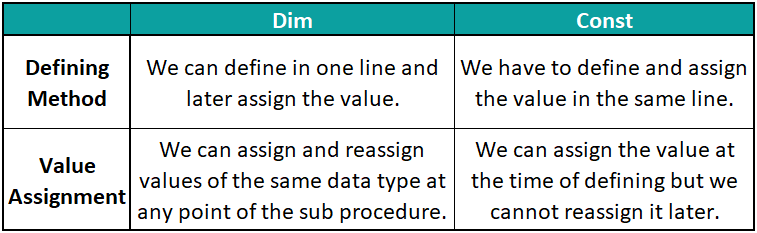2. Why use VBA Const instead of Macro?

VBA Const can be used inside or outside a macro. It cannot be a replacement for macro.

3. Why is VBA Const Not Working?

VBA Const will throw a compile error if we try to reassign a value after we define it initially in the procedure.

4. What data type is Const in VBA?

We can assign any data type to the VBA Const. The following are some of the common data types assigned to the VBA Const.
String
Boolean
Long
Int
Byte